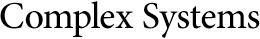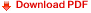## Logic, Explainability and the Future of UnderstandingStephen Wolfram
Founder and CEO
Wolfram Research, Inc.
s.wolfram@wolfram.com

#### Abstract

Logic is a foundation for many things. But what are the foundations of logic itself?

In symbolic logic, one introduces symbols like p and q to stand for statements (or “propositions”) like “this is an interesting essay”. Then one has certain “rules of logic”, like that, for any p and any q, NOT (p AND q) is the same as (NOT p) OR (NOT q).

Keywords: mathematical logic; automatic theorem proving

Cite this publication as:
S. Wolfram, “Logic, Explainability and the Future of Understanding,” Complex Systems, 28(1), 2019 pp. 1–40.
https://doi.org/10.25088/ComplexSystems.28.1.1